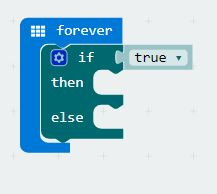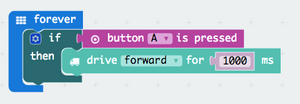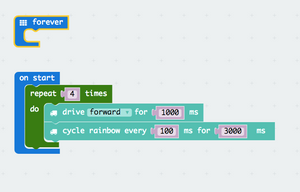GiggleBot - The Micro:Bit Robot
GiggleBot - The Micro:Bit Robot

# Coding Examples — microbit robot

## micro:bit Programming - Use the if then else statement in MakeCode

bbc microbit robot if then else if then else microbit robotIn this article we explore the "if then else" block in the MakeCode programming language.  This block is a logic block that extends the "if then" block.  We wrote an introduction to the "if then" block here.  In this example, we will show you how to program and if-then-else block and control a robot, helping the robot to decide if it should move forward or stop.

Read more →

## micro:bit Programming - Make an if statement with a robotWriting an if statement for the micro:bit is very easy.  Here we will show you how to program an if-statement with the micro:bit robot, using the GiggleBot robot as an example for programming.

Read more →

## micro:bit Programming - Make a For LoopIn this tutorial we'll go over how to make a "for loop" for our Micro:Bit. We will make the robot the GiggleBot robot move forward and shine some lights.

Read more →